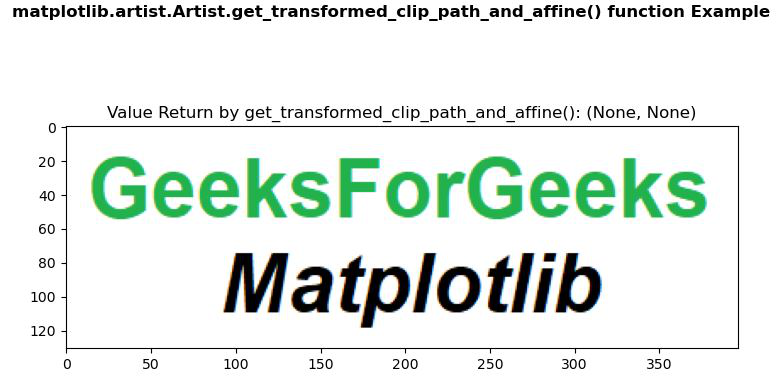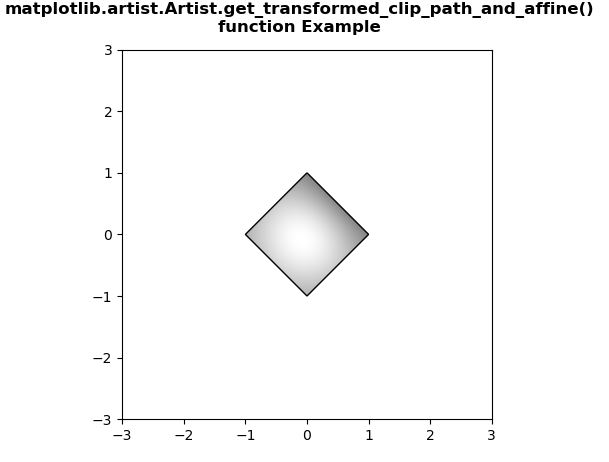# Matplotlib.artist.Artist.get_transformed_clip_path_and_affine() in Python

• Last Updated : 15 May, 2020

Matplotlib is a library in Python and it is numerical – mathematical extension for NumPy library. The Artist class contains Abstract base class for objects that render into a FigureCanvas. All visible elements in a figure are subclasses of Artist.

## Matplotlib.artist.Artist.get_transformed_clip_path_and_affine() Method

The get_transformed_clip_path_and_affine() method in artist module of matplotlib library is used to get the clip path with the non-affine part of its transformation applied, and the remaining affine part of its transformation.

Attention geek! Strengthen your foundations with the Python Programming Foundation Course and learn the basics.

To begin with, your interview preparations Enhance your Data Structures concepts with the Python DS Course. And to begin with your Machine Learning Journey, join the Machine Learning - Basic Level Course

Syntax: Artist.get_transformed_clip_path_and_affine(self)

Parameters: This method does not accepts any parameter.

Returns: This method return the clip path with the non-affine part of its transformation applied, and the remaining affine part of its transformation.

Below examples illustrate the matplotlib.artist.Artist.get_transformed_clip_path_and_affine() function in matplotlib:

Example 1:

Image Used:`# Implementation of matplotlib function``from` `matplotlib.artist ``import` `Artist ``import` `matplotlib.pyplot as plt ``import` `matplotlib.patches as patches ``import` `matplotlib.cbook as cbook ``      ` `   ` `with cbook.get_sample_data(``'image.PNG'``) as image_file: ``    ``image ``=` `plt.imread(image_file) ``      ` `fig, ax ``=` `plt.subplots() ``im ``=` `ax.imshow(image) ``patch ``=` `patches.Rectangle((``0``, ``0``), ``260``, ``200``,  ``                          ``transform ``=` `ax.transData) `` ` `# use of get_transformed_clip_path_and_affine() method``val ``=` `Artist.get_transformed_clip_path_and_affine(im)``ax.set_title(``"Value Return by get_transformed_clip_path_and_affine(): "` `             ``+` `str``(val))`` ` `fig.suptitle('matplotlib.artist.Artist.get_transformed_clip_path_and_affine() \``function Example', fontweight``=``"bold"``)`` ` `plt.show()`

Output:Example 2:

 `# Implementation of matplotlib function``from` `matplotlib.artist ``import` `Artist ``import` `numpy as np ``import` `matplotlib.cm as cm ``import` `matplotlib.pyplot as plt ``from` `matplotlib.path ``import` `Path ``from` `matplotlib.patches ``import` `PathPatch ``      ` `   ` `delta ``=` `0.025``   ` `x ``=` `y ``=` `np.arange(``-``3.0``, ``3.0``, delta) ``X, Y ``=` `np.meshgrid(x, y) ``   ` `Z1 ``=` `np.exp(``-``X``*``*``2` `-` `Y``*``*``2``) ``Z2 ``=` `np.exp(``-``(X ``-` `1``)``*``*``2` `-` `(Y ``-` `1``)``*``*``2``) ``Z ``=` `(Z1 ``-` `Z2) ``*` `2``      ` `path ``=` `Path([[``0``, ``1``], [``1``, ``0``], [``0``, ``-``1``], [``-``1``, ``0``], [``0``, ``1``]]) ``patch ``=` `PathPatch(path, facecolor ``=``'none'``) ``      ` `fig, ax ``=` `plt.subplots() ``ax.add_patch(patch) ``im ``=` `ax.imshow(Z, ``               ``interpolation ``=``'bilinear'``,  ``               ``cmap ``=` `cm.gray, ``               ``origin ``=``'lower'``, ``               ``extent ``=``[``-``3``, ``3``, ``-``3``, ``3``], ``               ``clip_path ``=` `patch, ``               ``clip_on ``=` `True``)`` ` `# use of get_transformed_clip_path_and_affine() method``val ``=` `Artist.get_transformed_clip_path_and_affine(im)``print``(``"Value Return by get_transformed_clip_path_and_affine(): "``)``for` `i ``in` `val: ``    ``print``(i) `` ` `fig.suptitle(``"""matplotlib.artist.Artist.get_transformed_clip_path_and_affine()``function Example"""``, fontweight``=``"bold")`` ` `plt.show()`

Output:```Value Return by get_transformed_clip_path_and_affine():
Path(array([[ 0.,  1.],
[ 1.,  0.],
[ 0., -1.],
[-1.,  0.],
[ 0.,  1.]]), None)
Affine2D(
[[ 82.66666667   0.         328.        ]
[  0.          61.6        237.6       ]
[  0.           0.           1.        ]])
```

My Personal Notes arrow_drop_up